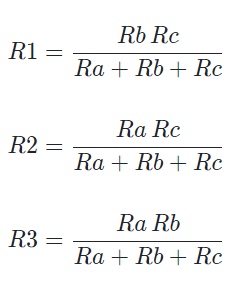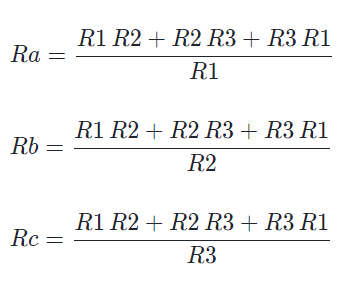# Delta Wye Calculator

## The Delta Wye transformation, also known as the Y-Δ or “delta-star” transformation because of its shapes of the circuits,   is a procedure used to simplify complex resistor networks.  This calculation transforms a wye configuration to a delta configuration or a delta configuration to a wye configuration, with the objective of facilitating circuit analysis.

DELTA
Resistor (RA)
Resistor (RB)
Resistor (RC)
WYE
Resistor (R1)
Resistor (R2)
Resistor (R3)

### Equations

Δ → Y transformation

Equations for transforming a Δ network into a Y network:Transforming from Δ to Y introduces one additional node.

Y → Δ transformation

Equations for transforming a Y network into a Δ network:Transforming from Y to Δ removes one node.

### Application

Delta and Wye configurations are used in three-phase power systems, and the Delta configuration appears in the bridge rectifier circuit that performs the first step in converting AC voltage to a stable DC voltage.